Electron. J. Diff. Eqns., Monograph 08, 2007, (101 pages).

### An algorithm for constructing Lyapunov functions Sigurdur Freyr Hafstein

Abstract:
In this monograph we develop an algorithm for constructing Lyapunov functions for arbitrary switched dynamical systems $$\dot{\mathbf{x}} = \mathbf{f}_\sigma(t,\mathbf{x})$$, possessing a uniformly asymptotically stable equilibrium. Let $$\dot{\mathbf{x}}=\mathbf{f}_p(t,\mathbf{x})$$, $$p\in \mathcal{P}$$, be the collection of the ODEs, to which the switched system corresponds. The number of the vector fields $$\mathbf{f}_p$$ on the right-hand side of the differential equation is assumed to be finite and we assume that their components $$f_{p,i}$$ are $$\mathcal{C}^2$$ functions and that we can give some bounds, not necessarily close, on their second-order partial derivatives. The inputs of the algorithm are solely a finite number of the function values of the vector fields $$\mathbf{f}_p$$ and these bounds. The domain of the Lyapunov function constructed by the algorithm is only limited by the size of the equilibrium's region of attraction. Note, that the concept of a Lyapunov function for the arbitrary switched system $$\dot{\mathbf{x}} = \mathbf{f}_\sigma(t,\mathbf{x})$$ is equivalent to the concept of a common Lyapunov function for the systems $$\dot{\mathbf{x}}=\mathbf{f}_p(t,\mathbf{x})$$, $$p\in\mathcal{P}$$, and that if $$\mathcal{P}$$ contains exactly one element, then the switched system is just a usual ODE $$\dot{\mathbf{x}}=\mathbf{f}(t,\mathbf{x})$$. We give numerous examples of Lyapunov functions constructed by our method at the end of this monograph.
DOI: https://doi.org/10.58997/ejde.mon.08

In this monograph we develop an algorithm for constructing Lyapunov functions for arbitrary switched dynamical systems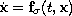, possessing a uniformly asymptotically stable equilibrium. Let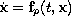,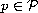, be the collection of the ODEs, to which the switched system corresponds. The number of the vector fields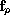on the right-hand side of the differential equation is assumed to be finite and we assume that their components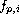are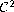functions and that we can give some bounds, not necessarily close, on their second-order partial derivatives. The inputs of the algorithm are solely a finite number of the function values of the vector fieldsand these bounds. The domain of the Lyapunov function constructed by the algorithm is only limited by the size of the equilibrium's region of attraction. Note, that the concept of a Lyapunov function for the arbitrary switched systemis equivalent to the concept of a common Lyapunov function for the systems,, and that if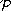contains exactly one element, then the switched system is just a usual ODE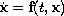. We give numerous examples of Lyapunov functions constructed by our method at the end of this monograph.

Submitted August 29, 2006. Published August 15, 2007.
Math Subject Classifications: 35J20, 35J25.
Key Words: Lyapunov functions; switched systems; converse theorem; piecewise affine functions.

Show me the PDF file (6694 KB), TEX file, and other files for this article.Sigurdur Freyr Hafstein School of Science and Engineering Reykjavik University Reykjavik, Iceland email: sigurdurh@ru.is